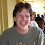## Tuesday, April 07, 2015

### Two cutoffs about Warings problem for cubes

Known:
1. All numbers except 23 can be written as the sum of 8 cubes
2. All but a finite number of numbers can be written as the sum of 7 cubes
3. There are an infinite number of numbers that cannot be written as the sum of 3 cubes(this you can prove yourself, the other two are hard, deep theorems).
Open: Find x such that:
1. All but a finite number of numbers can be written as the sum of x cubes.
2. There exists an infinite number of numbers that cannot be written as the sum of x-1 cubes.
It is known that 4 ≤ x ≤ 7

Lets say you didn't know any of this and were looking at empirical data.

1. If you find that every number ≤ 10 can be written as the sum of 7 cubes this is NOT interesting because 10 is too small.
2.  If you find that every number ≤ 1,000,000 except 23 can be written as the sum of 8 cubes this IS interesting since 1,000,000 is big enough that one thinks this is telling us something (though we could be wrong). What if you find all but 10 numbers (I do not know if that is true) ≤ 1,000,000 are the sum of seven cubes?
Open but too informal to be a real question: Find x such that
1. Information about sums-of-cubes for all numbers ≤ x-1 is NOT interesting
2. Information about sums-of-cubes for all numbers ≤ x IS interesting.
By the intermediate value theorem such an x exists. But of course this is silly. The fallacy probably relies on the informal notion interesting'. But a serious question: How big does x have to be before data about this would be considered interesting? (NO- I won't come back with what about x-1').

More advanced form: Find a function f(x,y) and constants c1 and c2 such that
1. If f(x,y) ≥ c1  then the statement all but y numbers ≤ x are the sum of 7 cubes is interesting.
2. If f(x,y) ≤  c2 then the statement all but y numbers ≤ x are the sum of 7 cubes is not interesting.
To end with a more concrete question: Show that there are an infinite number of numbers that cannot be written as the sum of 14 4th powers.

1.All integers except 23 and 239 are sums of eight cubes. https://projecteuclid.org/euclid.bams/1183502007

2.239 cannot be represented by the sum of 8 natural cubes, but can be represented by 8 integer cubes.
239 = 512 - 343 + 125 - 81 + 27 - 1

3.I think how interesting the data is depends on the data, not the size of the data. If the largest exception is N and you have results up to 2N, it's hard to say you have evidence that there are no further exceptions greater than 2N. But if the largest exception is N and you have results up to 1000N, that's interesting.

4.If we're allowing cubes of negative numbers, then 23 is representable as well.
23 = 27 - 8 + 1 + 1 + 1 + 1 (+ 0 + 0)

5.Let A be a random subset of integers with the same density as cubes: each natural n is included in A with probability n^(-2/3). Let Y(x) be a random variable indicating now many integers less than x cannot be presented as a sum of 7 elements of A. You statement "all but y numbers ≤ x are the sum of 7 cubes" becomes "interesting" if y is substantially different from EY(x). To make the word "interesting" more concrete, we can set up a limit in say 0.01, that is, the statement is interesting if and only if P(|Y(x)-EY(x)| > |y-EY(X)|) > 0.01. This indicate that with 99% chance there is some "interesting" structure distinguising additive properties of cubes from random sets with the same density. Once this structure is identified, it can be included into the definition of A (now it is a random set subject to this structure) and the process can be repeated.